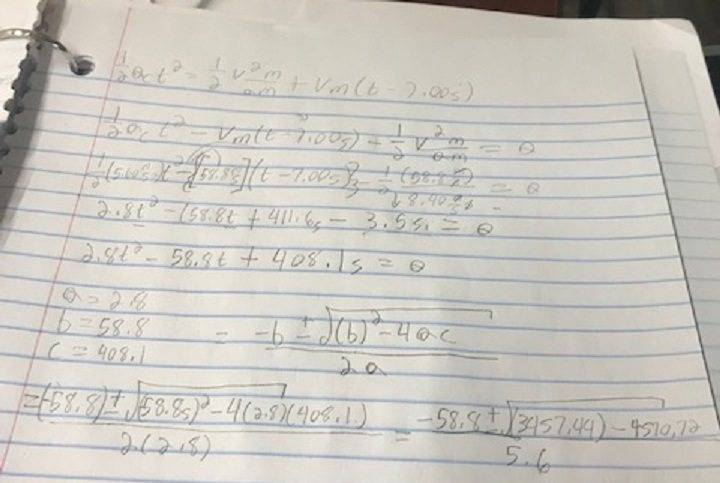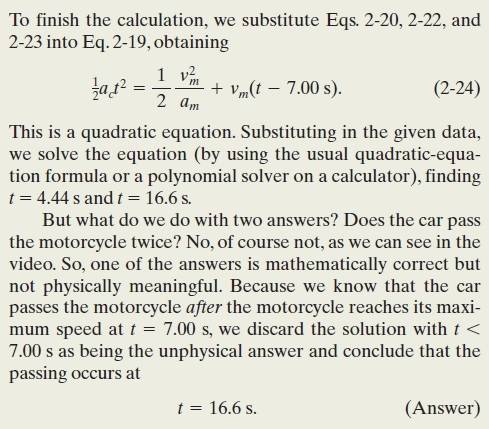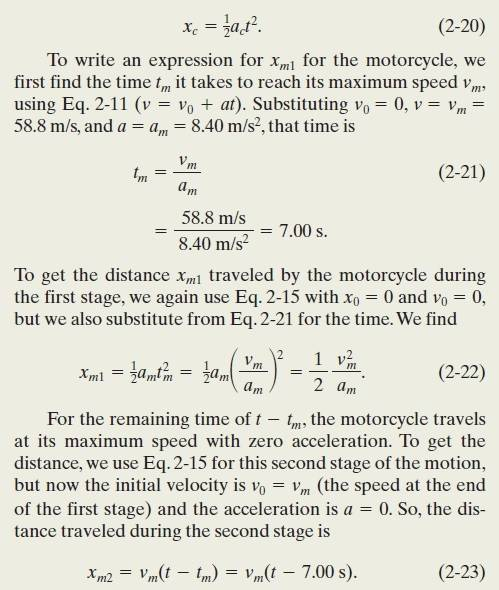# Quadratic equation help (Time when one vehicle passes another)

Homework Statement:
popular web video shows a jet airplane, a car, and a motorcycle
racing from rest along a runway. Initially
the motorcycle takes the lead, but then the jet takes the lead,
and finally the car blows past the motorcycle. Here let’s focus
on the car and motorcycle and assign some reasonable values
to the motion.The motorcycle first takes the lead because its
(constant) acceleration am = 8.40 m/s2 is greater than the car’s
(constant) acceleration ac = 5.60 m/s2, but it soon loses to the
car because it reaches its greatest speed vm =58.8 m/s before
the car reaches its greatest speed vc =106 m/s. How long does
the car take to reach the motorcycle?
Relevant Equations:
$$\frac{1}{2}ac{t^2} = \frac{1}{2}\frac{{{v^2}m}}{{am}} + (t - 7.00s)$$

((1/2)act^2=(1/2)(vm^2/am)+(t-7.00s)
Sample Problem 2.04 Drag race of car and motorcycle

I was following all the way up to using the quadratic equation for this problem...(please see img for a more detailed attempt at a solution.)

So I may have simplified incorrectly here:but I came up with

2.8t^2-(58.8)t+408.1=0

but when filling in

a=2.8
b=58.8
c=408.1

in the quadratic equation to find "t" The root would be negative. So I'm stuck. The answer to the example problem is 4.44 s and t 16.6 s which 16.6 would be the correct answer for t, I just am stuck at how they got these answers from the quadratic equation.

Last edited by a moderator:

kuruman
Homework Helper
Gold Member
In the final quadratic b = -58.8, but that does not fix the problem.

The problem is in your original equation that is dimensionally incorrect. The left side has units of length and the right side has units of time.

•Selectron09
Hi kuruman, thanks for your reply. Unfortunately that still makes the root in the quadratic equation negative because

$$\frac{{ - b \pm \sqrt {{{( - 58.8)}^2} - 4ac} }}{{2a}}$$

and I calculated -4(a)(c)= 4570.72 which would equal (-1113.28) under root.

kuruman
Homework Helper
Gold Member
The problem is in your original equation that is dimensionally incorrect. The left side has units of length and the right side has units of time. I don't know what it is supposed to represent, but you need to start with equations one for the position of the car at any time t and a second for the position of the motorcycle at any time t. Then say that there is a particular time ##t_c## at which the car catches up. At that time the two expressions for the positions must be equal.

•Selectron09
The equation is unlikely to be incorrect as it came from the textbook's example problem.haruspex
Homework Helper
Gold Member
2020 Award
The equation is unlikely to be incorrect as it came from the textbook's example problem.
You copied it incorrectly. Try again.

•Selectron09You copied it incorrectly. Try again.

Wouldn't you want to put it in the form = 0 before you put a,b,c into quadratic?

haruspex
Homework Helper
Gold Member
2020 Award
I don't understand why you have posted the textbook image again. The error is not there; the error is in the copy you made in post #1.

•Selectron09
I posted that so you would know what they are referring to by 2-20-2-23

haruspex
Homework Helper
Gold Member
2020 Award
Wouldn't you want to put it in the form = 0 before you put a,b,c into quadratic?
That is not it either.
However, looking at your handwritten working you may have used the correct version and only made the mistake in typing the Relevant Equation in post #1. Give me a moment to check further.

•Selectron09
That is not it either.
However, looking at your handwritten working you may have used the correct version and only made the mistake in typing the Relevant Equation in post #1. Give me a moment to check further.

Thankyou

I'm still new to using MathType for formatting help in Physics Forum and Math stack exchange.

haruspex
Homework Helper
Gold Member
2020 Award
I'm still new to using MathType for formatting help in Physics Forum and Math stack exchange.
Ok. The error you made in post#1 is that you left out a vm in the last term.
But I think your problem is that the two questions are not quite the same. Does the car reach the motorcycle while it is still accelerating or only after having reached its maximum speed?

•Selectron09
Ok. The error you made in post#1 is that you left out a vm in the last term.
But I think your problem is that the two questions are not quite the same. Does the car reach the motorcycle while it is still accelerating or only after having reached its maximum speed?

I believe the question is referring to the car reaching the motorcycle while it is still accelerating because the question asks "
How long does
the car take to reach the motorcycle?" So the car I believe must still be accelerating

haruspex
Homework Helper
Gold Member
2020 Award
I believe the question is referring to the car reaching the motorcycle while it is still accelerating because the question asks "
How long does
the car take to reach the motorcycle?" So the car I believe must still be accelerating
The question states:
" [the motorcycle] reaches its greatest speed vm =58.8 m/s before the car reaches its greatest speed vc =106 m/s."
vc > vm, so the car will eventually reach the motorcycle whether or not it has reached maximum speed.

•Selectron09
Thanks for that insight. I'll rework it in the morning here in the states.

SammyS
Staff Emeritus
Homework Helper
Gold Member
Problem Statement: popular web video shows a jet airplane, a car, and a motorcycle
racing from rest along a runway. Initially
the motorcycle takes the lead, but then the jet takes the lead,
and finally the car blows past the motorcycle. Here let’s focus
on the car and motorcycle and assign some reasonable values
to the motion.The motorcycle first takes the lead because its
(constant) acceleration am = 8.40 m/s2 is greater than the car’s
(constant) acceleration ac = 5.60 m/s2, but it soon loses to the
car because it reaches its greatest speed vm =58.8 m/s before
the car reaches its greatest speed vc =106 m/s. How long does
the car take to reach the motorcycle?
Relevant Equations: $$\frac{1}{2}ac{t^2} = \frac{1}{2}\frac{{{v^2}m}}{{am}} + (t - 7.00s)$$

((1/2)act^2=(1/2)(vm^2/am)+(t-7.00s)

Sample Problem 2.04 Drag race of car and motorcycle

I was following all the way up to using the quadratic equation for this problem...(please see img for a more detailed attempt at a solution.)

So I may have simplified incorrectly here:

View attachment 243816

but I came up with

2.8t^2-(58.8)t+408.1=0
As pointed out by others, your Relevant Equation(s) is incorrect. It's missing a vm

It should be: ##\quad \dfrac{1}{2}a_c {t^2} = \dfrac{1}{2}\dfrac{{v_m^2}}{{a_m}} + v_m(t - 7.00)##
(I also fixed the subscripts.)

The problem you are having with obtaining the correct solution has to do with an error in substituting values correctly. It's somewhat difficult to read from that image of your workings, but you neglected to square the ##v_m##.

•Selectron09
As pointed out by others, your Relevant Equation(s) is incorrect. It's missing a vm

It should be: ##\quad \dfrac{1}{2}a_c {t^2} = \dfrac{1}{2}\dfrac{{v_m^2}}{{a_m}} + v_m(t - 7.00)##
(I also fixed the subscripts.)

The problem you are having with obtaining the correct solution has to do with an error in substituting values correctly. It's somewhat difficult to read from that image of your workings, but you neglected to square the ##v_m##.
Thanks a lot!

kuruman
$$RHS=\frac{1}{2}\frac{{v_m^2}}{{a_m}} + v_m(t -\frac{v_m}{a_m} )=v_mt-\frac{v_m^2}{2a_m}.$$With its mix of symbols and numbers, the solution presented in the textbook may have contributed OP's difficulties. Note that the above expression can be used as an auxiliary kinematic equation describing the object's position as a function of time, but only after the object has reached the constant velocity part of its motion.
•